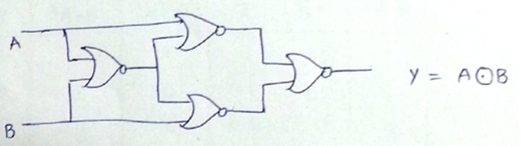# Universal Gates: NAND and NOR with Examples

Universal Logic Gates: In this tutorial, we will learn about universal logic gates (NAND and NOR gates), their trust tables, and examples. Submitted by Saurabh Gupta, on November 13, 2019

NAND and NOR Gates are known as Universal Logic Gates, because we can realize any logic circuit or gate only by using NAND or NOR gates single-handedly.

Although the logic circuit of any complexity can be realized using three basic gates i.e., AND, OR, NOT Gates, but they can also be realized using only a single type of gate, that is why sometimes NAND and NOR gates are called "universal building blocks". Thus, AOI logic can be converted into NAND or NOR logic.

## 1) NAND Gate

NAND Gate is a combination of two gates. It is an AND Gate followed by a NOT Gate where the output of AND Gate is inverted using a NOT Gate to get the final output. The logic operation for the NAND gate can be written as Y= A.B

Symbol and Truth Table for a two-input NAND Gate is: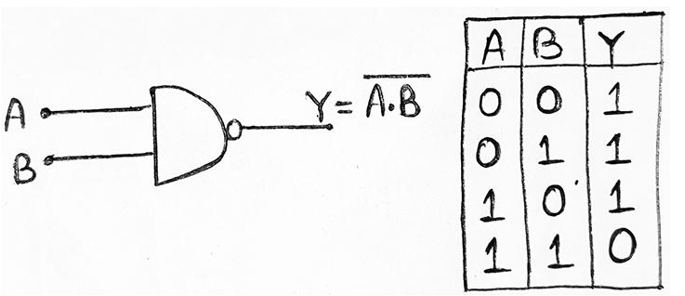## 2) NOR Gate

NOR Gate is also a combination of two gates namely, OR Gate followed by a NOT Gate where the output of OR Gate is inverted using a NOT Gate to get the final output. The logic operation of NOR Gate can be written as Y = A+B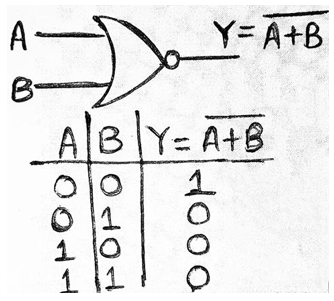## Universal Gates Examples

### Question 1

Realize the following gates by only using NAND Gates.

1. AND
2. OR
3. NOT
4. NOR
5. XOR
6. XNOR

1. AND Gate can be implemented using NAND Gate as: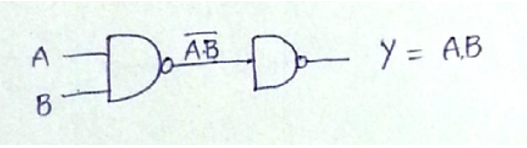2. OR Gate can be implemented using NAND Gate as: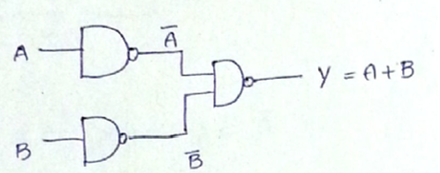3. NOT Gate can be implemented using NAND Gate as: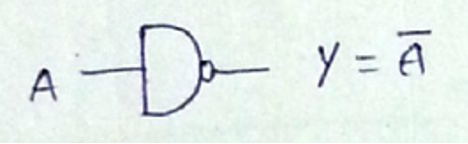4. NOR Gate can be implemented using NAND Gate as: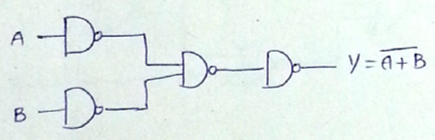5. XOR Gate can be implemented using NAND Gate as: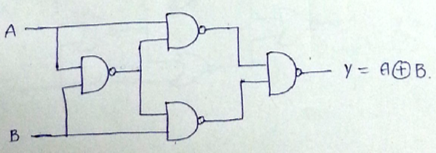6. XNOR Gate can be implemented using NAND Gate as: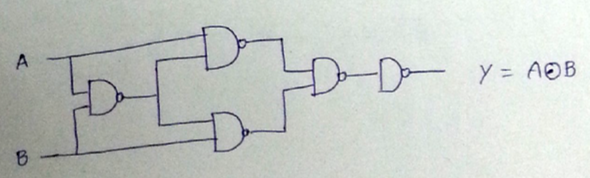### Question 2

Realize the following gates by only using NOR Gates.

1. AND
2. OR
3. NOT
4. NOR
5. XOR
6. XNOR

1. AND Gate can be implemented using NOR Gate as: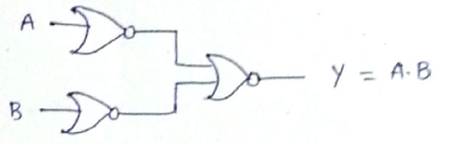2. OR Gate can be implemented using NOR Gate as: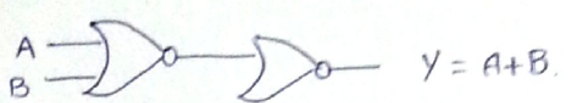3. NOT Gate can be implemented using NOR Gate as: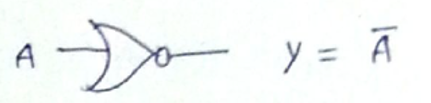4. NAND Gate can be implemented using NOR Gate as: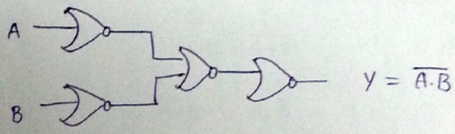5. XOR Gate can be implemented using NOR Gate as: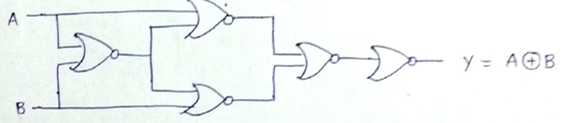6. XNOR Gate can be implemented using NOR Gate as: